# Analytical Reasoning (Test 2)

## Cat Entrance Exams : Data Interpretation And Logical Reasoning

| Home | | Cat Entrance Exams | | Data Interpretation And Logical Reasoning | | Analytical Reasoning |Analytical Reasoning
| Analytical Reasoning |
Q.1
Find the number of triangles in the given figure.A. 21
B. 23
C. 25
D. 27
Explaination / Solution:

The figure may be labelled as shown.The simplest triangles are ABL, BCD, DEF, FGP, PGH, QHI, JQI, KRJ and LRK i.e. 9 in number.

The triangles composed of two components each are OSG, SGQ, SPI, SRI, KSQ, KMS, FGH, JHI and JKL i.e. 9 in number.

There is only one triangle i.e. KSG which is composed of four components.

The triangles composed of five components each are NEI, ANI, MCG and KCO i.e. 4 in number.

The triangles composed of six components each are GMK and KOG i.e. 2 in number.

There is only one triangle i.e. AEI composed of ten components.

There is only one triangle i.e. KCG composed of eleven components.

Therefore, Total number of triangles in the given figure = 9 + 9+1 + 4 + 2+1 + 1 = 27.

Workspace
Report
Q.2
Find the number of triangles in the given figure.A. 10
B. 19
C. 21
D. 23
Explaination / Solution:

The figure may be labelled as shown.The simplest triangles are ABI, BIC, AIJ, CIJ, AHJ, CDJ, JHG, JDE, GJF and EJF i.e. 10 in number.

The triangles composed of two components each are ABC, BCJ, ACJ, BAJ, AJG, CJE and GJE i.e. 7 in number.

The triangles composed of four components each are ACG, ACE, CGE and AGE i.e. 4 in number.

Total number of triangles in the figure =10+ 7 + 4 = 21.

Workspace
Report
Q.3
Find the number of triangles in the given figure.A. 5
B. 6
C. 8
D. 10
Explaination / Solution:

The figure may be labelled as shown.The simplest triangles are AJF, FBG, GCH, HDI and IEJ i.e. 5 in number.

The triangles composed of three components each EBH, AIC, EFC, ADG and BJD i.e. 5 in number.

Thus, there are 5 + 5 = 10 triangles in the figure.

Workspace
Report
Q.4
Find the minimum number of straight lines required to make the given figure.A. 9
B. 11
C. 15
D. 16
Explaination / Solution:

The figure may be labelled as shown.The horizontal lines are DE, FH, IL and BC i.e. 4 in number.

The slanting lines are AC, DO, FN, IM, AB, EM and HN i.e. 7 in number.

Thus, there are 4 + 7 = 11 straight lines in the figure.

Workspace
Report
Q.5
Find the number of triangles in the given figure.A. 10
B. 12
C. 14
D. 16
Explaination / Solution:

The figure may be labelled as shown.The simplest triangles are ABJ, ACJ, BDH, DHF, CIE and GIE i.e. 6 in number.

The triangles composed of two components each are ABC, BDF, CEG, BHJ, JHK, JKI and CJI i.e. 7 in number.

There is only one triangle JHI which is composed of four components.

Thus, there are 6 + 7 + 1 = 14 triangles in the given figure.

Workspace
Report
Q.6

Find the number of triangles in the given figure.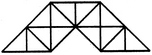A. 23
B. 27
C. 29
D. 31
Explaination / Solution:

The figure may be labelled as shown.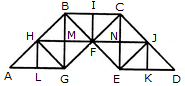The simplest triangles are AHL, LHG, GHM, HMB, GMF, BMF, BIF, CIF, FNC, CNJ, FNE, NEJ, EKJ and JKD i.e. 14 in number.

The triangles composed of two components each are AGH, BHG, HBF, BFG, HFG, BCF, CJF, CJE, JEF, CFE and JED i.e. 11 in number.

The triangles composed of four components each are ABG, CBG, BCE and CED i.e. 4 in number.

Total number of triangles in the given figure = 14 + 11 + 4 = 29.

Workspace
Report
Q.7

Find the number of triangles in the given figure.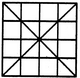A. 36
B. 40
C. 44
D. 48
Explaination / Solution:

The figure may be labelled as shown.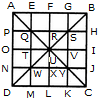The simplest triangles are APQ, AEQ, QTU, QRU, BGS, BHS, RSU, SUV, TUW, UWX, NWD, WDM, UVY, UXY, JCY and YKC i.e. 16 in number.

The triangles composed of two components each are QUW, QSU, SYU and UWY i.e. 4 in number.

The triangles composed of three components each are AOU, AFU, FBU, BIU, UIC, ULC, ULD and OUD i.e. 8 in number.

The triangles composed of four components each are QYW, QSW, QSY and SYW i.e. 4 in number.

The triangles composed of six components each are AUD, ABU, BUC and DUC i.e. 4 in number.

The triangles composed of seven components each are QMC, ANY, EBW, PSD, CQH, AGY, DSK and BJW i.e. 8 in number.

The triangles composed of twelve components each are ABD, ABC, BCD and ACD i.e. 4 in number.

Thus, there are 16 + 4 + 8 + 4 + 4 + 8 + 4 = 48 triangles in the figure.

Workspace
Report
Q.8

Find the number of triangles in the given figure.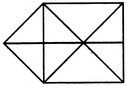A. 15
B. 16
C. 17
D. 18
Explaination / Solution:

The figure may be labelled as shown.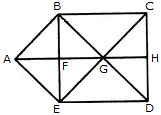The simplest triangles are ABF, BFG, BCG, CGH, GHD, GED, EFG and AFE i.e. 8 in number.

The triangles composed of two components each are ABG, BGE, AGE, ABE and GCD i.e. 5 in number.

The triangles composed of three components each are BCD, CDE, BED and BCE i.e. 4 in number.

Thus, there are 8 + 5 + 4 = 17 triangles in the figure.

Workspace
Report
Q.9

Find the number of triangles in the given figure.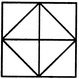A. 8
B. 10
C. 12
D. 14
Explaination / Solution:

The figure may be labelled as shown.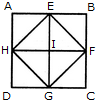The simplest triangles are AEH, EHI, EBF, EFI, FGC, IFG, DGH and HIG i.e. 8 in number.

The triangles composed of two components each are HEF, EFG, HFG and EFG i.e. 4 in number.

Thus, there are 8 + 4 = 12 triangles in the figure.

Workspace
Report
Q.10

Find the number of triangles in the given figure.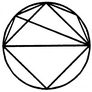A. 8
B. 10
C. 11
D. 12
Explaination / Solution:

The figure may be labelled as shown.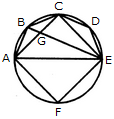The simplest triangles are ABG, BCG, CGE, CDE, AGE and AEF i.e. 6 in number.

The triangles composed of two components each are ABE, ABC, BCE and ACE i.e. 4 in number.

There are 6 + 4 = 10 triangles in the figure.

Workspace
Report